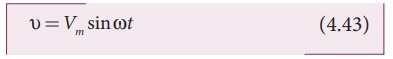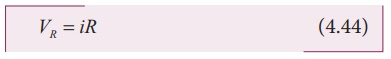Home | | Physics 12th Std | AC circuit containing pure resistor

# AC circuit containing pure resistor

Consider a circuit containing a pure resistor of resistance R connected across an alternating voltage source.

AC circuit containing pure resistorConsider a circuit containing a pure resistor of resistance R connected across an alternating voltage source (Figure 4.45). The instantaneous value of the alternating voltage is given byAn alternating current i flowing in the circuit due to this voltage, develops a potential drop across R and is given byKirchoffâ€™s loop rule (Refer section 2.4) states that the algebraic sum of potential differences in a closed circuit is zero. For this resistive circuit,where Vm/R = Im ,  the peak value of alternating current in the circuit. From equations (4.43) and (4.45), it is clear that the applied voltage and the current are in phase with each other in a resistive circuit. It means that they reach their maxima and minima simultaneously. This is indicated in the phasor diagram (Figure 4.46). The wave diagram also depicts that current is in phase with the applied voltage (Figure 4.46).Tags : Phasor diagram, Circuit Diagram, Formula | Alternating Current (AC) , 12th Physics : Electromagnetic Induction and Alternating Current
Study Material, Lecturing Notes, Assignment, Reference, Wiki description explanation, brief detail
12th Physics : Electromagnetic Induction and Alternating Current : AC circuit containing pure resistor | Phasor diagram, Circuit Diagram, Formula | Alternating Current (AC)